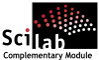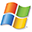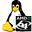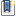ATOMS : Condition Number details

# Condition Number

Evaluates the condition number of functions.Details
Version
0.1
A more recent valid version with binaries for Scilab 5.2 exists: 0.2
Author
Michael Baudin
Owner Organization
DIGITEO
Maintainer
Michael Baudin
Category
Dependency
Creation Date
December 14, 2010
Source created on
Scilab 5.2.x
Binaries available on
Scilab 5.2.x:Windows 64-bitWindows 32-bitLinux 64-bitLinux 32-bitMacOSX
Install command
`--> atomsInstall("condnb")`
Report a bugDescription
```            Purpose
----

These functions provides the condition number of various elementary functions.
Their goal is to evaluate the potential numerical difficulty of evaluating
numerically a function at a given point.
We may use this module while testing a given function.

The toolbox is based on macros.

To get an overview of the functions in this module, use :

help condnb_overview

This module uses the apifun module.

Features
--------

The following is a list of the current functions :
* condnb_condnum : Computes the empirical condition number of the function f at
point x.
* condnb_acoscond : Computes the condition number of the acos function.
* condnb_asincond : Computes the condition number of the asin function.
* condnb_atancond : Computes the condition number of the atan function.
* condnb_coscond : Computes the condition number of the cos function.
* condnb_erfcond : Computes the condition number of the erf function.
* condnb_erfinvcond : Computes the condition number of the erfinv function.
* condnb_expcond : Computes the condition number of the exp function.
* condnb_logcond : Computes the condition number of the log function.
* condnb_plotcond : Plots the condition number of a function.
* condnb_powcond : Computes the condition number of the pow function.
* condnb_sincond : Computes the condition number of the sin function.
* condnb_sqrtcond : Computes the condition number of the square root function.
* condnb_sumcond : Computes the condition number of the sum function.
* condnb_tancond : Computes the condition number of the tan function.

Forge
-----

http://forge.scilab.org/index.php/p/condnb/
```Files (2)Source code archive
```
```OS-independent binary for Scilab 5.2.x
```Mise à jour du fichier de Description.
```News (0)Comments (0)Leave a comment
You must register and log in before leaving a comment.Email notifications
Send me email when this toolbox has changes, new files or a new release.